×

Search anything:

# Symbol Table in Compiler

#### Compiler Design Software EngineeringGet this book -> Problems on Array: For Interviews and Competitive Programming

In this article, we have explored Symbol Table in Compiler in depth. We presented 3 different approaches to implement Symbol Table using Linear List, Tree and Hash Table data structures.

1. Symbol Table in Compiler
2. Possible Implementations Techniques
• (i) Linear Lists
• (ii) Trees
• (iii) Hash Table
• Hash Function
• Implementing a Hash Function
• Another Hash Function
• Resolving collisions through Open Addressing
• Separate Lists Chaining
3. Implementing Symbol Tables
4. Uses of Symbol Table
5. Symbol Table Format
6. Tree structure representation of scope information
7. Block structures and non block structure storage allocation
8. Symbol Table Class Definition
9. Symbol Table Class Implementation
10. Symbol Table Class Definition
11. Initialize and Clear a Symbol Table
12. Lookup a name in a Symbol Table
13. Insert a name into a Symbol Table
14. Symbol Insertion Representation
15. Lookup and then Insert a name

Pre-requisites:

# Symbol Table in Compiler

A symbol table is a data structure employed by a language translator, like a compiler or interpreter, within which each identifier in a program's source code is connected with information about its declaration or presence in the source code, like its type, scope level, and infrequently its position.

A symbol table is a significant data structure used in a compiler that correlates characteristics with program identifiers. The analysis and synthesis stages employ symbol table information to verify that used identifiers have been specified, to validate that expressions and assignments are semantically accurate, and to build intermediate or target code.

A symbol table's basic operations are allocate, free, insert, lookup, set attribute and get attribute. The allocate operation assigns an empty symbol table. To remove all records and free the storage of a symbol table, free operation is used. The insert operation as the name suggests inserts a name in a symbol table and return a pointer to its entry. The lookup function searches for a name and returns a pointer to its entry. The set attribute and the get attribute associate an attribute with a given entry and get an attribute associated with a given respectively. Other procedures might be added based on the needs. A delete operation, for instance, removes a previously entered name.

# Possible Implementations Techniques

There are three data structures used to implement symbol table:

1. Linear List
2. Binary Search Tree
3. Hash Table

## (i) Linear Lists

It is the simplest and most straightforward method of implementing data structures. To store names and their accompanying information, we use a single array. New names are added to the list in the order that they appear. To add a new name, we must first check the list to ensure that it does not already exist. If it is not present, it should be added; otherwise, an error notice, such as Multiply declared name, is shown. When the name is identified, the accompanying information may be found in the words that follow. To obtain information about a name, we search from the beginning of the array up to the location indicated by the AVAILABLE pointer, which denotes the start of the array's empty half. If we get to the AVAILABLE pointer without finding NAME, we have a problem — we've used an undefined name.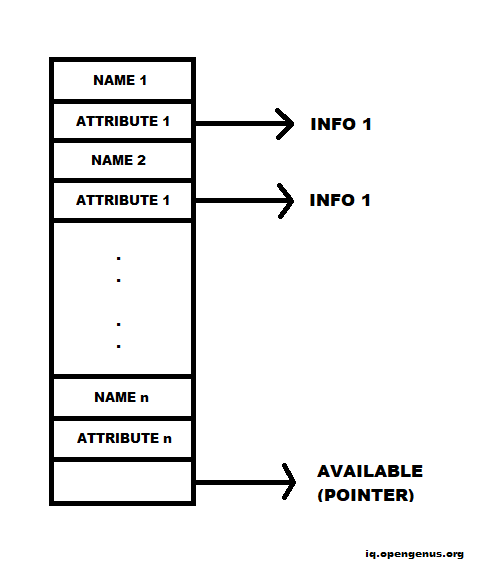On average, we will search n/2 names to get information on the NAME. As a result, the cost of an inquiry is proportional to n.

One advantage of list organization is that simple compilers use the least amount of space feasible.

The list is broken into two sections:

• Unordered list: Used for a modest number of variables.
• Ordered list: In this, the cost of insertion is high, but the process is straightforward.

## (ii) Trees

It is a more efficient method of organizing symbol tables. Each record now has two link fields, LEFT and RIGHT. The procedure below is used to find NAME in a binary search tree where x is originally a reference to the root.

``````1. while x ≠ null do
2. if NAME = NAME(x) then      /* NAME found take action on success*/
3. else if NAME < NAME(x) then
x:=LEFT(x)         /* visit left child*/
5. else                        /* NAME (p)<NAME*/
X:= RIGHT(x)               /*visit right child*/
``````

## (iii) Hash Table

Hashing table technique is suitable for searching and hence it is implemented in compiler.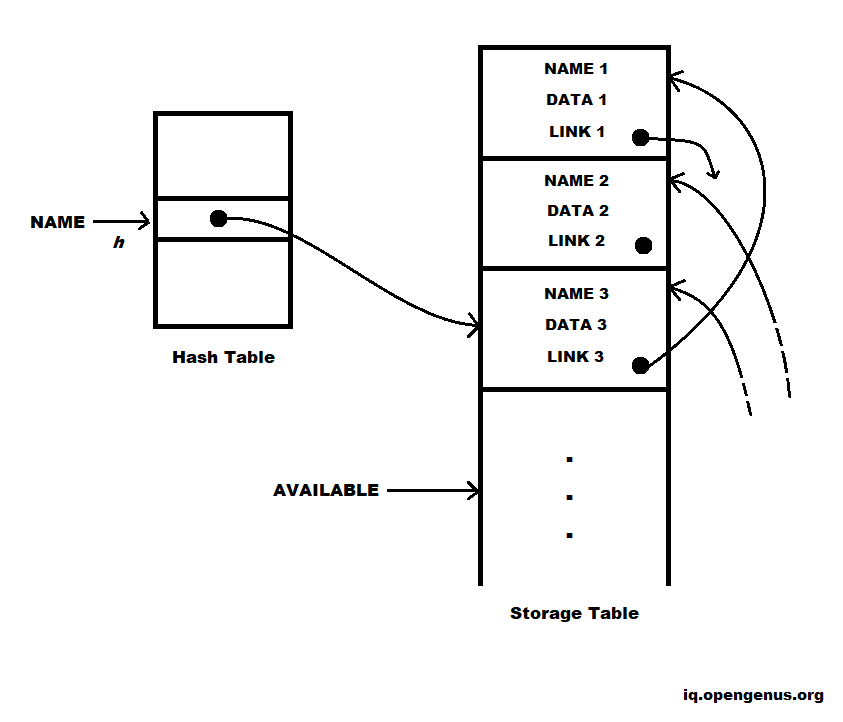The fundamental hashing schema is depicted in the diagram above. Two tables are used: a hash table and a storage table.

The hash table is made up of k words with numbers 0,1,2,...,k-1.
These words are pointers to the heads of k distinct linked lists in the storage table (some lists may be empty). Each symbol table record appears on one of these lists.

We use NAME as a hash function h such that h(NAME) is an integer between 0 and k-1 to determine if NAME is in the symbol table.

## Hash Function

The hash function is the mapping between an item and the place in the hash table where that item resides. The hash function takes a collection item and returns an integer in the range of slot names from 0 to m-1.

Example:

Assume that we have the set of integer items 59, 28, 93, 18, 77 and 31.
Consider m=11 (number of slots of hash table)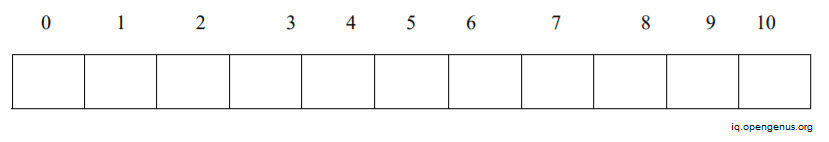Suppose we want to insert the above elements within the slots by using formula:

h(item) % m
59 % 11 = 4
28 % 11 = 6
93 % 11 = 5
18 % 11 = 7
77 % 11 = 0
31 % 11 = 9
After inserting above elements the hash table looks as shown below: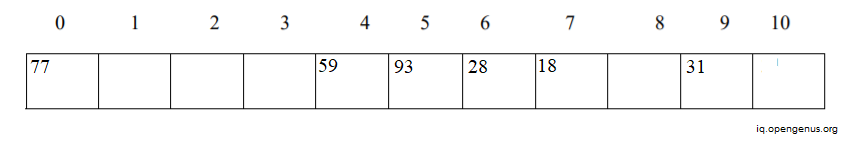## Implementing a Hash Function

``````// Hash string s
// Hash value = (sn-1 + 16(sn-2 + .. + 16(s1+16s0)))
// Return hash value (independent of table size)

unsigned hash(char* s) {
unsigned hval = 0;
while (*s != ’\0’) {
hval = (hval << 4) + *s;
s++;
}
return hval;
}
``````

### Another Hash Function

``````// Treat string s as an array of unsigned integers
// Fold array into an unsigned integer using addition
// Return hash value (independent of table size)

unsigned hash(char* s) {
unsigned hval = 0;
while (s!=0 && s!=0 && s!=0 && s!=0){
unsigned u = *((unsigned*) s);
hval += u; s += 4;
}
if (s == 0) return hval;
hval += s;
if (s == 0) return hval;
hval += s<<8;
if (s == 0) return hval;
hval += s<<16;
return hval;
}
``````

### Resolving collisions through Open Addressing

When h(name1) = h(name2) and name1 ≠ name2, a collision occurs. Because the name space of identifiers is significantly bigger than the table size, collisions are unavoidable. So, how does one handle collisions?

• If h(name) is taken, try h2(name), h3(name), and so on. This method is known as Open Addressing.
• h2(name) is the same as h(name) + 1 mod. TableSize
• h3(name) is the same as h(name) + 2 mod. TableSize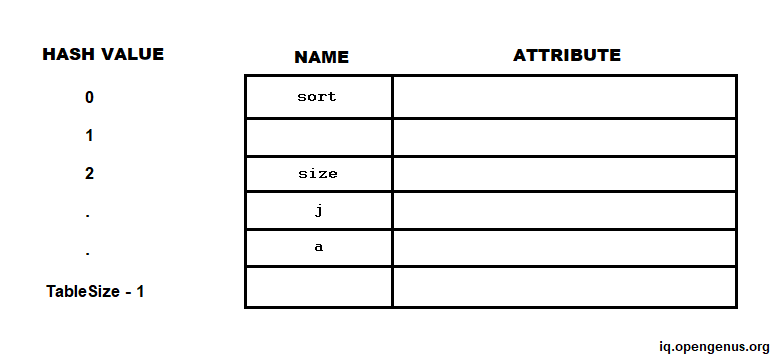### Separate Lists Chaining

• As the array fills, collisions become more common - performance suffers
• Table size is a problem - dynamically raising table size is challenging.

Chaining using different lists is an alternative to open addressing.

• The hash table is an array of pointers to linked lists called buckets
• The number of identifiers is no longer limited by the table size
• When the number of identifiers exceeds TableSize, lookup is O(n/TableSize).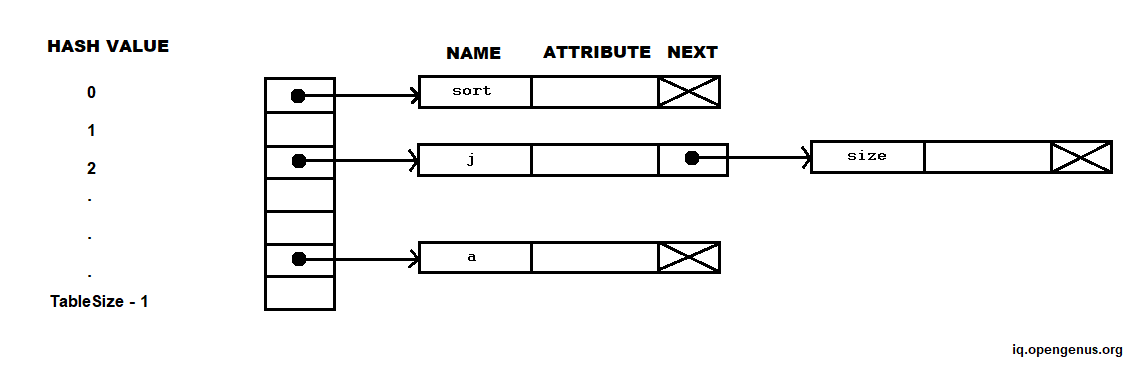# Implementing Symbol Tables

Symbol tables can be implemented in a variety of ways, but the most key distinction is how scopes are handled. This can be accomplished using either a permanent (or functional) data structure or an imperative (or destructively updated) data structure.
A persistent data structure has the property of not being destroyed by any action on it. In theory, whenever an operation modifies a data structure, a new updated copy is created, leaving the old structure unaltered.

Only one copy of the symbol table exists in the imperative approach, therefore explicit actions are necessary to save the information required to restore the symbol table to a prior state. An auxiliary stack can be used to do this. When a name is rewritten, the old binding of that name is recorded (pushed) on the auxiliary stack.

A marker is put on the auxiliary stack when a new scope is entered. The bindings on the auxiliary stack (down to the marker) are used to reconstruct the old symbol table when the scope is terminated. In the process, the bindings and the marker are popped off the auxiliary stack, restoring it to the state it was in before the scope was entered.

# Uses of Symbol Table

An object file will have a symbol table containing the externally visible identifiers it includes. A linker will utilize these symbol tables to resolve any unresolved references during the linking of distinct object files.

A symbol table may only exist during the translation process, or it may be included in the process' output for later use, for as during an interactive debugging session or as a resource for creating a diagnostic report during or after program execution.

Many tools use the symbol table to examine what addresses have been assigned to global variables and known functions while reverse engineering an executable. Tools will have a tougher time determining addresses or understanding anything about the program if the symbol table has been stripped or cleaned away before being translated into an executable.

A compiler must do various tasks while accessing variables and dynamically allocating memory, which is why the extended stack model requires the symbol table.

# Symbol Table Format

A symbol table is a data structure that gathers scope information and keeps information about identifiers. Each item in the symbol table has the following properties: Name, Kind, Type, and others.
The following is the format of the symbol table: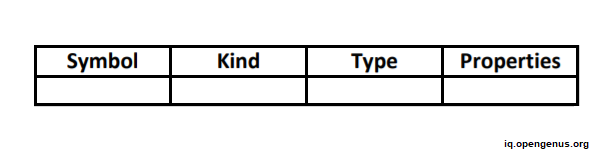The following is the scope nesting mirrored hierarchy of symbol tables: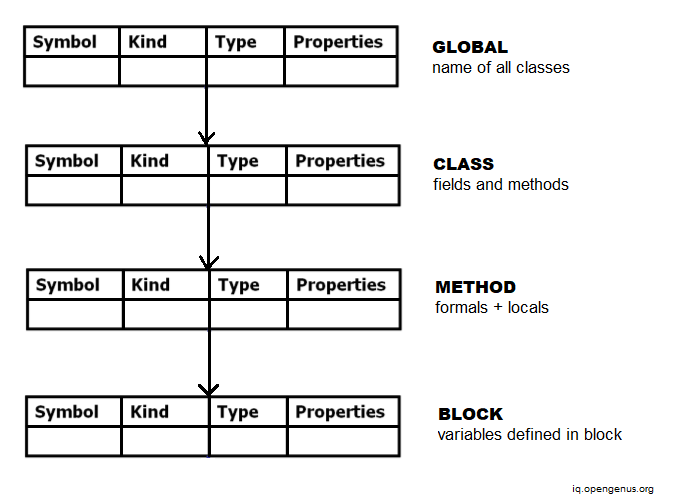Example:
Consider the following program.

``````class Foo {
int value;
int test() {
int b = 3;
return value + b;
}
void setValue(int c) {
value = c;
{    int d = c;
c = c + d;
value = c;
}
}
}

class Bar {
int value;
void setValue(int c) {
value = c;
}
}
``````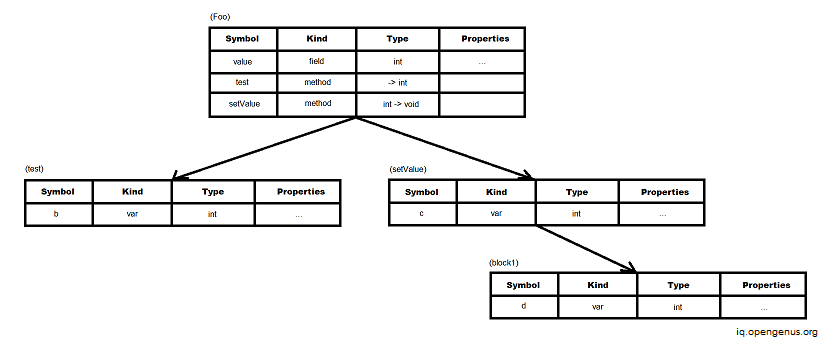### Tree structure representation of scope information

Scope information describes the declaration of identifiers as well as the parts of the program where each identifier is permitted to be used. Variables, functions, objects, and labels are some examples of identifiers.

Semantic Rules for Scopes:

The following are the main principles for scopes:

1. Use an identifier only if it is defined in the enclosing scope.
2. Do not declare the same kind of identifier with identical names more than once in the same lexical scope.

When the scope information is presented in a hierarchical fashion, a tree structure representation is formed. This format was created to address the disadvantage of the sequential representation being sluggish in looking for the necessary identifier.

The following is an example of how scope information is represented in a symbol table: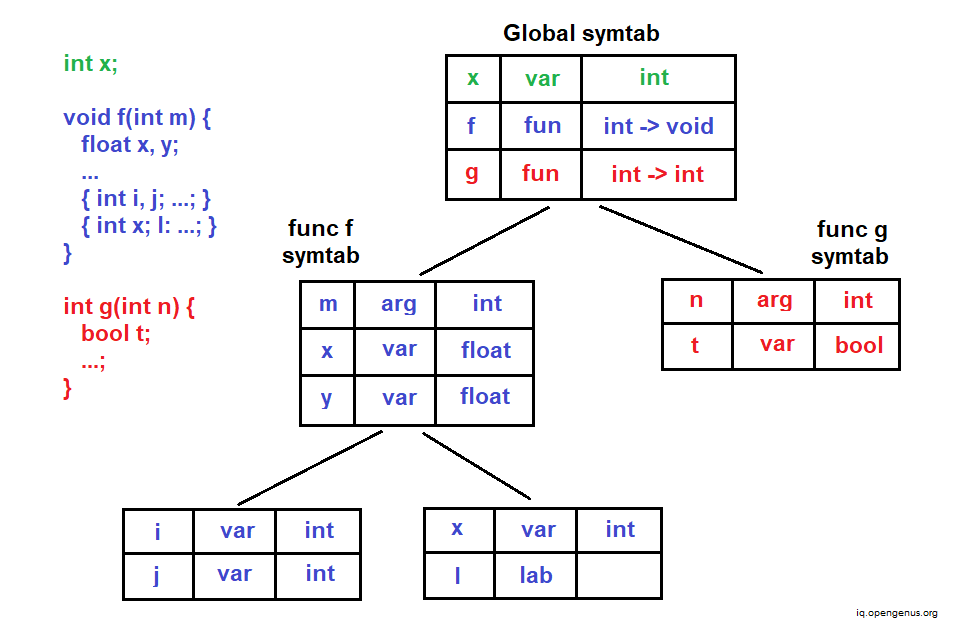The hierarchical structure of symbol tables addresses the problem of resolving name clashes automatically (identifiers with the same name and overlapping scopes).

### Block structures and non block structure storage allocation

The scoping rules of block structured languages are supported by block structured symbol tables. In general, storage can be allocated for two sorts of data variables:

1. Local Data
2. Non-Local Data

Local data is handled by activation records, whereas non-local data is handled by scope information.
Block organized storage can be allocated using either static or lexical scope. Dynamic scope can be used to allocate non-block structured storage.

# Symbol Table Class Definition

``````class Symbol {
friend class Table;
public:
Symbol(char* s);
~Symbol();
const char* id();
Symbol* nextinlist();
Symbol* nextinbucket();
.
.
.
private:
char* name;
Symbol* list;
Symbol* next;
.
.
.
};
``````

# Symbol Table Class Implementation

``````Symbol::Symbol(char* s) {
name = new char[strlen(s)+1];
strcpy(name,s);
next = list = 0;
}

Symbol::~Symbol() {
delete [] name;
name = 0;
next = list = 0;
}

const char* Symbol::id() {return name;}
Symbol* Symbol::nextinbucket() {return next;}
Symbol* Symbol::nextinlist() {return list;}
``````

# Symbol Table Class Definition

``````const unsigned HT_SIZE = 1021;
class Table {
public:
Table();
Symbol* clear();
Symbol* lookup(char*s);
Symbol* lookup(char*s,unsigned h);
Symbol* insert(char*s,unsigned h);
Symbol* lookupInsert(char*s);
Symbol* symlist() {return first;}
unsigned symbols(){return count;}
.
.
.
private:
Symbol* ht[HT_SIZE];
Symbol* first;
Symbol* last;
unsigned count;
};
``````

# Initialize and Clear a Symbol Table

``````Table::Table() {
for (int i=0; i<HT_SIZE; i++) ht[i] = 0;
first = last = 0;
count = 0;
}

Symbol* Table::clear() {
Symbol* list = first;
for (int i=0; i<HT_SIZE; i++) ht[i] = 0;
first = last = 0;
count = 0;
return list;
}
``````

# Lookup a name in a Symbol Table

``````Symbol* Table::lookup(char* s) {
unsigned h = hash(s);
return lookup(s,h);
}

Symbol* Table::lookup(char* s, unsigned h) {
unsigned index = h % HT_SIZE;
Symbol* sym = ht[index];
while (sym != 0) {
if (strcmp(sym->name, s) == 0) break;
sym = sym->next;
}
return sym;
}
``````

# Insert a name into a Symbol Table

``````Symbol* Table::insert(char* s, unsigned h) {
unsigned index = h % HT_SIZE;
Symbol* sym = new Symbol(s);
sym->next = ht[index];
ht[index] = sym;
if (count == 0) { first = last = sym; }
else {
last -> list = sym;
last = sym;
}
count++;
return sym;
}
``````

# Symbol Insertion Representation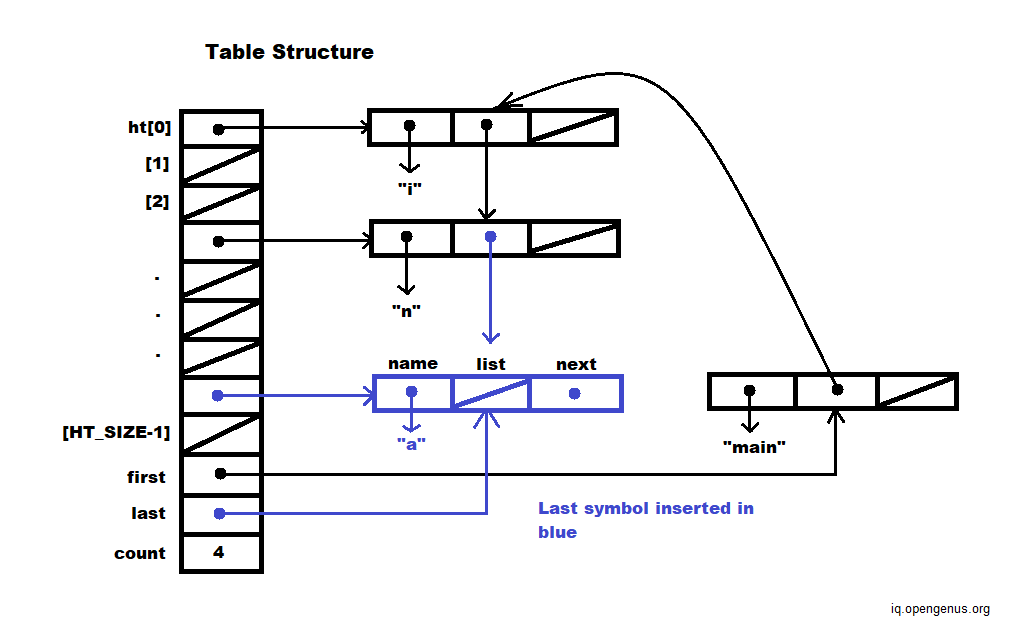# Lookup and then Insert a name

``````Symbol* Table::lookupInsert(char* s) {
unsigned h = hash(s);
Symbol* sym;
sym = lookup(s,h);
if (sym == 0) {
sym = insert(s,h);
}
return sym;
}
``````

With this article at OpenGenus, you must have the complete idea of Symbol Table in Compiler.#### Harshita Singh

Harshita Singh is pursuing her B. Tech in CSE from Harcourt Butler Technical University, Kanpur (2023). She is an Intern at OpenGenus.

Symbol Table in Compiler